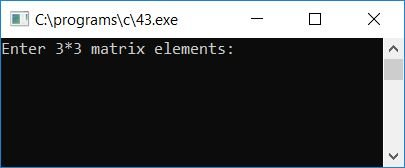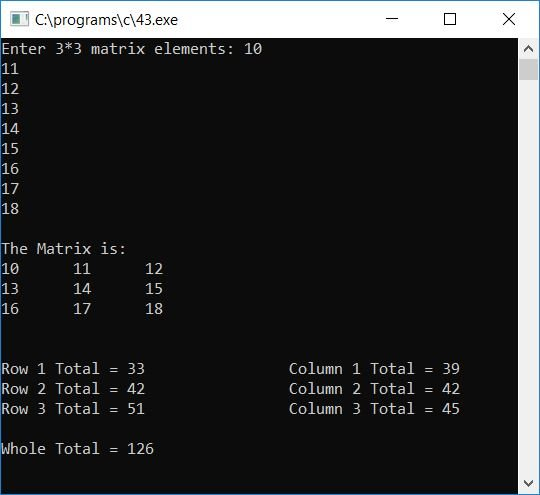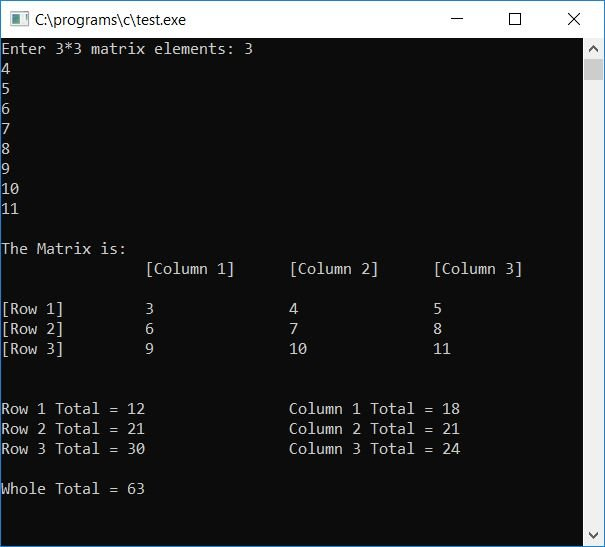# C Program to Print the Sum of Each Row and Column of a Given Matrix

In this tutorial, we will learn about how to create a program in C that will ask the user to enter any 3*3 matrix as input and print the sum of all elements present in each row and column along with its whole total. Here is the program:

```#include<stdio.h>
#include<conio.h>
int main()
{
int mat, i, j, rowtot, coltot, tot=0;
printf("Enter 3*3 matrix elements: ");
for(i=0; i<3; i++)
{
for(j=0; j<3; j++)
{
scanf("%d", &mat[i][j]);
}
}
printf("\nThe Matrix is:\n");
for(i=0; i<3; i++)
{
for(j=0; j<3; j++)
{
printf("%d\t",mat[i][j]);
}
printf("\n");
}
printf("\n");
for(i=0; i<3; i++)
{
rowtot=0;
coltot=0;
for(j=0; j<3; j++)
{
rowtot = rowtot + mat[i][j];
coltot = coltot + mat[j][i];
tot = tot + mat[i][j];
}
printf("\nRow %d Total = %d\t\t", i+1, rowtot);
printf("Column %d Total = %d", i+1, coltot);
}
printf("\n\nWhole Total = %d", tot);
getch();
return 0;
}```

The program was written in the Code::Blocks IDE; therefore, after a successful build and run, here is the sample run. This is the first snapshot of the sample run:Supply any 3*3 matrix element and press the ENTER key to see the output. At output, you will see the matrix, then the row-wise row total and the column-wise column total, then the whole total of the matrix elements as shown in the second snapshot of the sample run:Here are some of the main steps used in the above program:

• Receive any 3*3 matrix elements, which is a total of 9 numbers, as 3*3 or 9 elements for the matrix.
• Now display the matrix as output.
• To find the row total and column total for each row and column of the given matrix, follow these steps:
• Create a for loop that starts from 0 to 3, as the matrix we have taken from the user is 3*3.
• Inside the for loop, initialize 0 to any two variables, say rowtot and coltot, that will hold the row and column totals.
• Create another for loop that starts from 0 to 3 again.
• Inside this for loop, calculate the sum of row elements and column elements one by one.
• That is, if i is equal to zero, then inside the inner for loop, the loop variable j is set to 0.
• Therefore, at first run, rowtot will hold rowtot + mat[i][j] or 0 + mat, that is, 0 will be added to the very first element of the given matrix, and coltot will hold coltot + mat[j][i], that is, 0 will be added to the very first element of the given matrix. The first element of any matrix is the first element of the first row and the first column.
• And rowtot will hold rowtot + mat[i][j] or mat + mat on the second run.As previously, rowtot held the value of the first matrix element; therefore, we have replaced rowtot with mat, which will be added to the second element of the given matrix, and coltot will hold mat + mat, which will be added to the fourth element of the given matrix. The fourth element will be the second element in the first column.
• Continue the same process until the last element of the given matrix.
• After coming out of the inner for loop or before continuing to the outer for loop, print the value of the row total and column total.
• In this way, the program will print the sum of all three elements present in each row and column.
• The total for all 9 elements will be calculated and printed at the end of the program, after both the for loops have been exited.

Here is the modified version of the above program. The question is: write a program to read a value in a matrix and print its value along with its row total, column total, and whole total. Here we have displayed the matrix along with its row number and column number.

```#include<stdio.h>
#include<conio.h>
int main()
{
int mat, i, j, rowtot, coltot, tot=0;
printf("Enter 3*3 matrix elements: ");
for(i=0; i<3; i++)
{
for(j=0; j<3; j++)
{
scanf("%d", &mat[i][j]);
}
}
printf("\nThe Matrix is:\n");
printf("\t\t[Column 1]\t[Column 2]\t[Column 3]\n\n");
for(i=0; i<3; i++)
{
printf("[Row %d]\t\t", i+1);
for(j=0; j<3; j++)
{
printf("%d\t\t",mat[i][j]);
}
printf("\n");
}
printf("\n");
for(i=0; i<3; i++)
{
rowtot=0;
coltot=0;
for(j=0; j<3; j++)
{
rowtot = rowtot + mat[i][j];
coltot = coltot + mat[j][i];
tot = tot + mat[i][j];
}
printf("\nRow %d Total = %d\t\t", i+1, rowtot);
printf("Column %d Total = %d", i+1, coltot);
}
printf("\n\nWhole Total = %d", tot);
getch();
return 0;
}```

Here is the final snapshot of the sample run of the above program:C Quiz

« Previous Program Next Program »